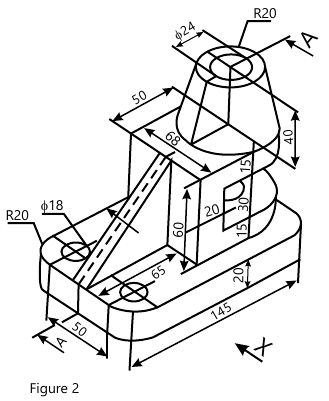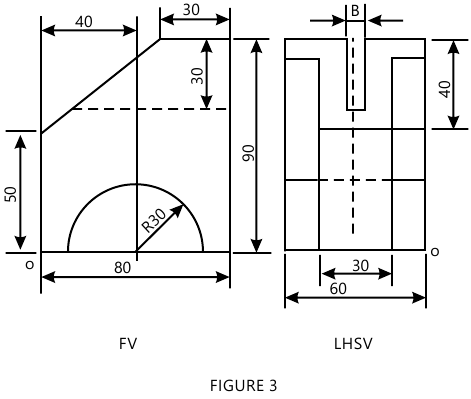MORE IN Engineering Drawing
MU First Year Engineering (Semester 2)
Engineering Drawing
December 2012
Total marks: --
Total time: --
INSTRUCTIONS
(1) Assume appropriate data and state your reasons
(2) Marks are given to the right of every question
(3) Draw neat diagrams wherever necessary

1 Figure shows FV & TV of the bracket. Draw the following views:-
(a) Sectional FV along A-A.
(b) Top view.
(c) R.H.S.V.
Insert at least 8 major dimensions.
15 M

2 Figure shows a pictorial view of a machine part. Draw
(a) Sectional FV along the arrow X (section A-A).
(b) Top view.
(c) L.H.S.V.
Insert at least 10 major dimensions.15 M

3 (a) The top view and the front view of a line AB measures 70 mm and 58 mm respectively. The line AB is inclined at an angle of 35° to the HP. The end A is 15 mm above the HP and 12 mm in front of the VP. The other end B is also in the first quadrant. Draw the projections of line AB and find its true length and true inclination with the VP.
8 M
3 (b) The distance of a focus from the directrix is 50 mm. A point moves in such a way that the eccentricity is equal to 3/2. Draw the locus of the point and name the curve. Also draw tangent and normal at any point on the curve.
7 M

4 A pentagonal pyramid 35 mm base edges and 70 mm height is resting on HP. With one of its triangular surface perpendicular to HP and parallel to VP, draw its projections.
15 M

5 (a) A hexagonal pyramid of 25 mm edge of the base and axis 50 mm long is resting on its triangular face in the HP. with the axis parallel to the VP. It is cut by a section plane perpendicular to HP and inclined at 30° to VP and passing through a point on the axis 20 mm from the base. Draw the TV, sectional FV and true shape of the section when the apex is removed.
12 M
5 (b) Draw neat, proportionate free hand sketches of the following:-
3 M

6 A cone of diameter base 90 mm and height 90 mm stands vertically on its base in HP. A semicircular hole of diameter 60 mm is cur through the cone, the axis of the hole is horizontal and intersects the axis of cone is 35 mm above the base of the cone. The flat face of the hole contains the aaxis of the cone and is perpendicular to VP. Draw the development of the cone with hole.
15 M

7 (a) Draw neat, proportionate free hand sketches of the following:-
(i) Square Nut.
(ii) Eye Foundation Bolt.
3 M
7 (b) Figure shows two views of an object. Draw its isometric view.12 M

More question papers from Engineering Drawing